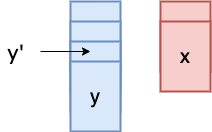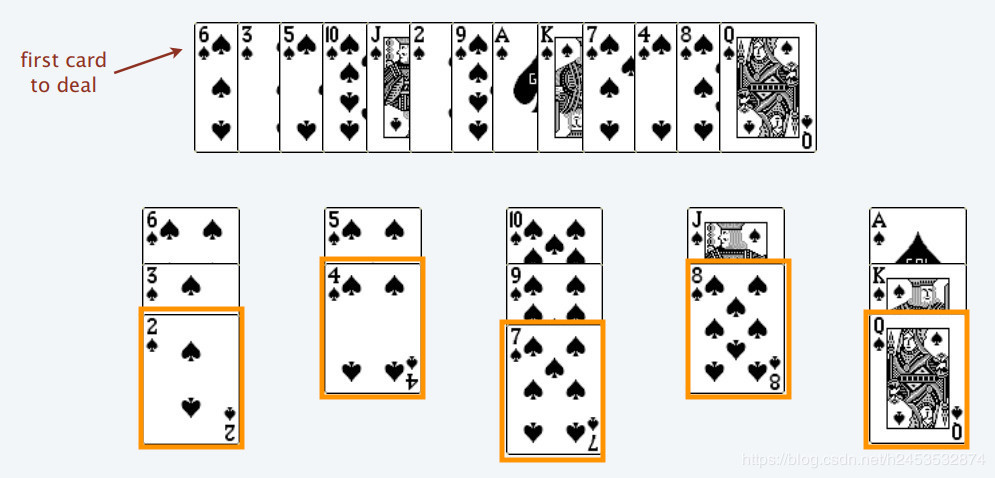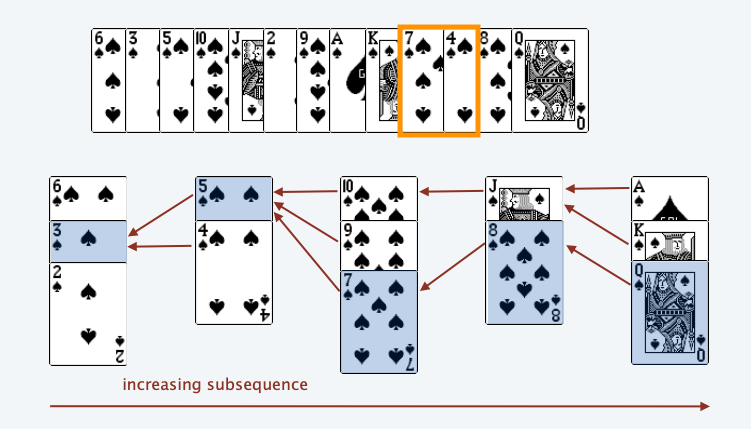# Sixian Li

## Longest Increasing Subsequence And Patience Game(Part1)

Algorithm

This part focuses on the idea behind the solution. The actual implementation will be covered in Part2.

## Problem

Given an unsorted array of integers, find the length of longest increasing subsequence. Example from LeetCode:

The $O(n^2)$ dynamic programming solution is quite straightforward. But I’m surprised to see the relationship between the card game Patience and LIS.

## Patience

Rules

1. You can only place a lowered-valued(or equal-valued) card on top of a higher-valued card, e.g., 2 on top of 3. Note Ace is NOT 1 here.

2. You can form a new pile and put your card onto it.

To understand why it’s related to LIS, we need to make two observations.

### Observation1

For any legal strategy, $\#\text{piles}$ $\geq$ length of any increasing subsequenceClaim: Any increasing subsequence can use at most one card from each pile.

#### Proof

Assume we used $y$ and $y'$ from this pile. Since $y$ is on top of $y'$, $y \le y’$.

If $y = y’$, they can’t form an increasing sequence. “increasing” is different from “non-decreasing”.

If $y \lt y’$, the only way to use both cards in an increasing sequence is to place $y'$ after $y$ in that sequence. But $y$ is on top of $y'$, so $y'$ comes before $y$ in the original array.

For example, [y', y] = [5, 2], 5 -> 2 is not an IS.

Thus, you can’t form an IS with more than one card from a pile $\Rightarrow$ length of any IS is at most $\#\text{piles}$.

### Greedy algorithm

Idea: put the new card on the leftmost pile possible, i.e., the first pile from left to right, such that $\text{new card} \le \text{top card of the pile}$.

If we play the game with this strategy, we will notice that top cards form an IS(increasing sequence). For example,2 4 7 8 Q is an IS.

Let’s prove this.

### Observation2

Using the greedy strategy, top cards of piles increase from left to right at any time of the game

#### ProofAssume $x \lt y$, so we don’t have an IS. We have two cases:

1. $y$ comes before $x$

Then $x$ must be placed on top of $y$ by the algorithm. Contradiction.

2. $y$ comes after $x$

Since $x$ is put into the red pile, $x \gt y'$ or it’ll be in the blue pile by our greedy algorithm. $y$ is on top of $y'$, so $y \le y'$. But we assumed $x \lt y$, so $x \lt y \le y'$ which contradicts $x\gt y'$.

With these two observations, we can go head and prove the duality.

## Duality

### Lenght of LIS = #piles using the greedy strategy

Let the length of LIS be $L$, and $P$ be the $\#\text{piles}$ created by the greedy algorithm. For an inequality, we have to show $“\geq”$ and $“\leq”$ directions.

1. $L \leq P$

This follows directly from $\#\text{piles}\geq$ length of nay IS in observation 1. LIS is an IS, so $L \leq P$.

1. $L \geq P$

From observation 2, we know top cards of piles increase from left to right at any time of the game using this algorithm.

Note that the top cards are in general not an increasing subsequence — Berkeley notes

In this case, the top cards are 2 4 7 8 Q, but 4 comes after 7 in the original deck, so 4 -> 7 is not an IS.To keep track of a valid IS, at the time of insertion of a card $c$, we keep a pointer from $c$ to the current top card to the left of $c$. For example, when 5 is inserted, 3 is the top card on its left, so there’s an arrow from 5 to 3.

Assume $P \gt L$, let the top card on the $i\text{th}$ pile be $a_i$. Since we have $P$ piles, we can follow the pointers starting at $a_p$ to get $a_1\leftarrow…\leftarrow a_{p-1} \leftarrow a_p$. Note that from left to right, $a_1 \lt a_2 \lt \cdots \lt a_p$. Thus, we get an IS of length $P$.

But we claimed the length of the longest IS is $L$. $P \gt L$ contraditcts that, so $P \leq L \ \square$

## Reference

Longest Increasing Subsequence

Patience Sorting